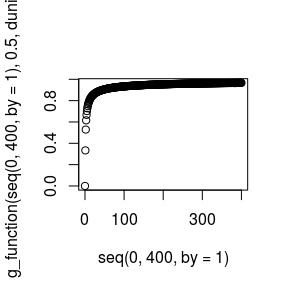# Kendall random walks

## Simulate and plot

# uzupelnic fig_captions, zeby dalo sie je zrobic
library(kendallRandomWalks)
kendall_rws <- simulate_kendall_rw(10, 100, runif, 0.25)
kendall_rws
#> Simulations of Kendall random walk
#> Number of simulations:  10
#> Length of a single simulation:  100
#> Step distribution:  runif
#> Alpha parameter:  0.25
plot(kendall_rws)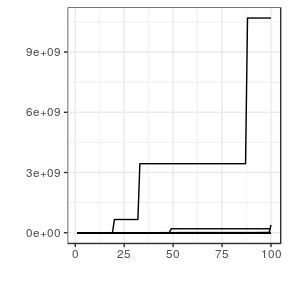plot(simulate_kendall_rw(10, 100, rnorm, 0.76), level = 300)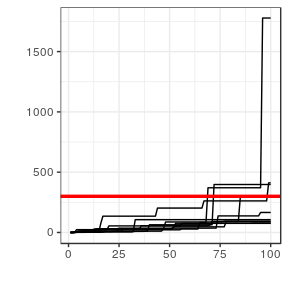Symmetric

kendall_rws_sym <- simulate_kendall_rw(10, 100, rnorm, 0.76, T)
kendall_rws_sym
#> Simulations of Kendall random walk
#> Number of simulations:  10
#> Length of a single simulation:  100
#> Step distribution:  rnorm
#> Alpha parameter:  0.76
plot(kendall_rws_sym)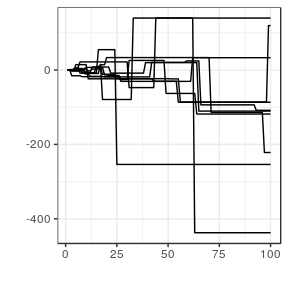## Barrier crossing

kendall_rws2 <- simulate_kendall_rw(1000, 100, runif, 0.25)
ladder_moments <- ladder_moment(kendall_rws2, 1000)
ladder_moments
#> Mean of the distribution:  14.917
#> Standard deviation of the distribution:  9.39374
#> Number of observations:  1000
#> Times the level was not crossed:  0
#> Quantiles of the distribution:
#>   0%  10%  20%  30%  40%  50%  60%  70%  80%  90% 100%
#>    3    5    7    9   11   13   15   17   21   27   70
plot(ladder_moments)
#> stat_bin() using bins = 30. Pick better value with binwidth.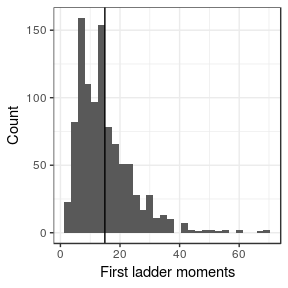ladder_heights <- ladder_height(kendall_rws2, 2000)
ladder_heights
#> Mean of the distribution:  2197744
#> Standard deviation of the distribution:  42066190
#> Number of observations:  1000
#> Times the level was not crossed:  0
#> Quantiles of the distribution:
#>           0%          10%          20%          30%          40%
#> 2.018395e+03 2.521659e+03 3.200712e+03 4.128353e+03 5.517956e+03
#>          50%          60%          70%          80%          90%
#> 8.734957e+03 1.413979e+04 2.315729e+04 4.960864e+04 1.663590e+05
#>         100%
#> 1.260329e+09
plot(ladder_heights)
#> stat_bin() using bins = 30. Pick better value with binwidth.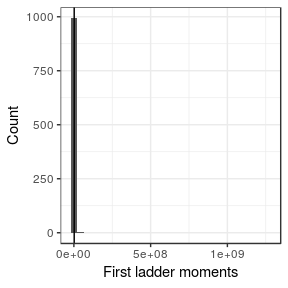Exact first ladder moments distribution with $$G(a)$$ computed numerically.

y <- seq(10, 10000, 50)
ladders <- sapply(y,
function(x)
ladder_moment_pmf(10, x, 0.5, pnorm, dnorm))
plot(y, ladders)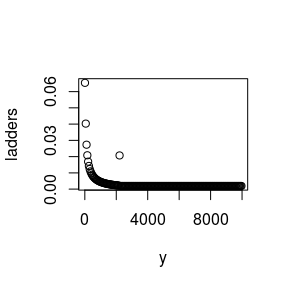y <- seq(2000, 2200, 1)
plot(y, g_function(y, 0.1, dnorm))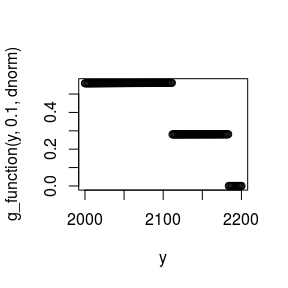plot(seq(0, 400, by = 1), g_function(seq(0, 400, by = 1), 0.5, dunif))